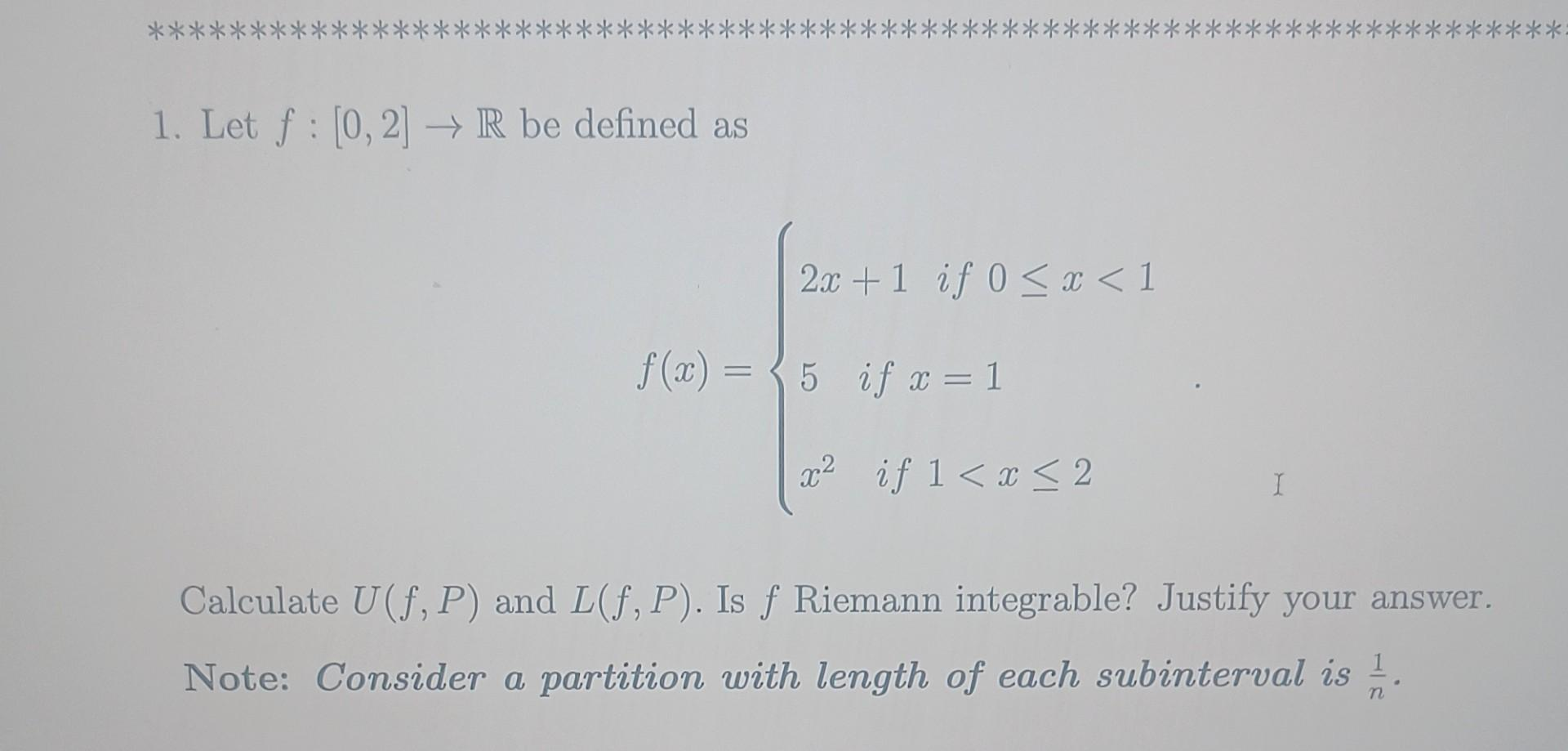# (Solved): 1. Let f:[0,2]R be defined as f(x)=2x+1if0x<15ifx=1x2if1<x ...1. Let be defined as Calculate and . Is Riemann integrable? Justify your answer. Note: Consider a partition with length of each subinterval is .

We have an Answer from Expert